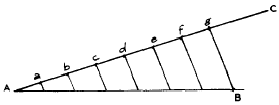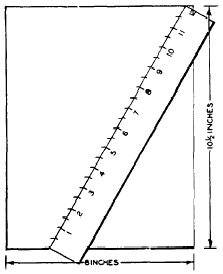Chapter 18 Geometric constructions and solid figuresCustom SearchCHAPTER 18 GEOMETRIC CONSTRUCTIONS AND SOLID FIGURES Many ratings in the Navy involve work which requires the construction or subdivision of geometric figures. For example, materials must be cut into desired shapes, perpendicular lines must be drawn, etc. In addition to these skills, some Navy ratings require the ability to recognize various solid figures and calculate their volumes and surface areas. CONSTRUCTIONS From the standpoint of geometry, a CONSTRUCTION may involve either the process of building up a figure or that of breaking down a figure into smaller parts. Some typical constructions are listed as follows: 1. Dividing a line into equal segments. 2. Erecting the perpendicular bisector of a line. 3. Erecting a perpendicular at any point on a line. 4. Bisecting an angle. 5. Constructing an angle. 6. Finding the center of a circle. 7. Constructing an ellipse. EQUAL DIVISIONS ON A LINE A line may be divided into any desired number of equal segments by the method shown in figure 18-1.Figure 18-1.-Dividing a line into equal segments. Suppose that line AB (fig. 18-1) is to be divided into seven equal segments. Draw line AC at any convenient angle with AB and mark off seven spaces of some convenient length, say l/2 inch, on it. Extend AC, if necessary, in order to get seven intervals of the chosen length on it. This produces the points a, b, c, d, e, f, and g, as shown in figure 18-1. Draw a line from g to B, and then draw lines parallel to gB, starting at each of the points a, b, c, d, e, and f. The segments of AB cut off by these lines are equal in length. It is frequently necessary to rule a predetermined number of lines on a blank sheet of material. This may be done by a method based on the foregoing discussion. For example, sup 18-2 is to be divided into 24 equal spaces. The 12-inch ruler is laid across the paper at an angle, in such a way that the ends of theFigure 18-2.-Ruling equal spaces on a sheet of paper  ruler coincide with the top and bottom edges of the paper. There are 24 spaces, each 1/2 inch wide, on a la-inch ruler. Therefore, we mark the paper beside each l/a-inch division marker on the ruler. After removing the ruler, we draw a line through each of the marks on the paper, parallel to the top and bottom edges of the paper. PERPENDICULAR BISECTOR OF A LINE To bisect a line or an angle means to divide it into two equal parts. A line may be bisected satisfactorily by measurement, or by a geo does not reach the full length of the line, proceed as follows: 1. Starting at one end, measure about half the length of the line and make a mark. 2. Starting at the other end, measure exactly the same distance as before and make a second mark. 3. The bisector of the line lies halfway between these two marks. The geometric method of bisecting a line is not dependent on measurement. It is based upon the fact that all points equally distant from the ends of a straight line lie on the perpendicular bisector of the line. Bisecting a line geometrically requires the use of a mathematical compass, which is an instrument for drawing circles and comparing distances. If a line AB is to be bisected as in figure 18-3, the compass is opened until the distance between its points is more than half as long as AB. Then a short arc is drawn above the approximate center of the line and another below, using A as the center of the arcs’ circle. (See fig. 18-3.) Two more short arcs are drawn, one above and one below the approximate center of line AB, this time using B as the center of the arcs’ circle. The two arcs above line AB are extended until they intersect, forming point C, and the two arcs below line AB intersect to form point D. The line joining point C and point D is the perpendicular bisector of line AB.Integrated Publishing, Inc. - A (SDVOSB) Service Disabled Veteran Owned Small Business